# Network security¶

In the early days, data networks were mainly used by researchers and security was not a concern. A few users were connected and capable of using the network. Almost all the devices attached to the network were openly accessible and users were trusted. As the utilization of the networks grew, security concerns started to appear. In universities, researchers and professors did not always trust their students and required some forms of access control. On standalone computers, the common access control mechanism is the password. A username is assigned to each user and when this user wants to access the computer, he or she needs to provide his/her username and his/her password. Most passwords are composed of a sequence of characters. The strength of the password is function of the difficulty of guessing the characters chosen by each user. Various guidelines have been defined on how to select a good password 1. Some systems require regular modifications of the passwords chosen by their users.

When the first computers were attached to data networks, applications were developed to enable them to access to remote computers through the network. To authenticate the remote users, these applications have also relied on usernames and passwords. When a user connects to a distant computer, she sends her username through the network and then provides her password to confirm her identity. This authentication scheme is presented in the time sequence diagram below.Note

Alice and Bob

Alice and Bob are the first names that are used in examples for security techniques. They first appeared in a seminal paper by Diffie and Hellman [DH1976]. Since then, Alice and Bob are the most frequently used names to represent the users who interact with a network. Other characters such as Eve or Mallory have been added over the years. We will explain their respective roles later.

## Threats¶

When analyzing security issues in computer networks, it is useful to reason about the capabilities of the attacker who wants to exploit some breach in the security of the network. There are different types of attackers. Some have generic capabilities, others are specific to a given technology or network protocol. In this section, we discuss some important threats that a network architect must take into account.

The first type of attacker is called the passive attacker. A passive attacker is someone able to observe and usually store the information (e.g. the packets) exchanged in a given network or subset of it (e.g. a specific link). This attacker has access to all the data passing through this specific link. This is the most basic type of attacker and many network technologies are vulnerable to such attacks. In the above example, a passive attacker could easily capture the password sent by Alice and reuse it later to be authenticated as Alice on the remote computer. This is illustrated in the figure below where we do not show anymore the DATA.req and DATA.ind primitives but only the messages exchanged. Throughout this chapter, we will always use Eve as a user who is able to eavesdrop the data passing in front of her.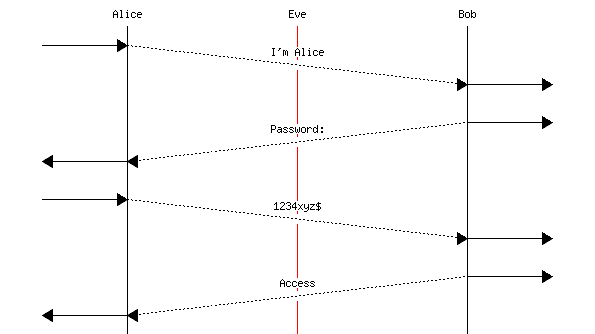In the above example, Eve can capture all the packets exchanged by Bob and Alice. This implies that Eve can discover Alice’s username and Alice’s password. With this information, Eve can then authenticate as Alice on Bob’s computer and do whatever Alice is authorized to do. This is a major problem from a security point of view. To prevent this attack, Alice should never send her password in clear over a network where someone could eavesdrop the information. In some networks, such as an open wireless network, an attacker can easily collect all the data sent by a particular user. In other networks, this is a bit more complex depending on the network technology used, but various software packages exist to automate this process. As will be described later, the best approach to prevent this type of attack is to rely on cryptographic techniques to ensure that passwords are never sent in clear.

Note

Pervasive monitoring

In the previous example, we have explained how Eve could capture data from a particular user. This is not the only attack of this type. In 2013, based on documents collected by Edward Snowden, the press revealed that several governmental agencies were collecting lots of data on various links that compose the global Internet [Greenwald2014]. Thanks to this massive amount of data, these governmental agencies have been able to extract lots of information about the behavior of Internet users. Like Eve, they are in a position to extract passwords, usernames and other privacy sensitive data from all the packets that they have captured. However, it seems that these agencies were often more interested in various meta data, e.g. information showing with whom a given user communicates than the actual data exchanged. These revelations have shocked the Internet community and the Internet Engineering Task Force that manages the standardization of Internet protocols has declared in RFC 7258 that such pervasive monitoring is an attack that need to be countered in the development of new protocols. Several new protocols and extensions to existing ones are being developed to counter these attacks.

Eavesdropping and pervasive monitoring are not the only possible attacks against a network. Another type of attacker is the active attacker. In the literature, these attacks are often called Man in the middle or MITM attacks. Such attacks occur when one user, let us call him Mallory, has managed to configure the network so that he can both capture and modify the packets exchanged by two users. The simplest scenario is when Mallory controls a router that is on the path used by both Alice and Bob. For example, Alice could be connected to a WiFi access router controlled by Mallory and Bob would be a regular server on the Internet.As Mallory receives all the packets sent by both Bob and Alice, he can modify them at will. For example, he could modify the commands sent by Alice to the server managed by Bob and change the responses sent by the server. This type of attack is very powerful and sometimes difficult to counter without relying on advanced cryptographic techniques.

The last type of attack that we consider in this introduction are the Denial of Service or DoS attacks. During such an attack, the attacker generates enough packets to saturate a given service and prevent it from operating correctly. The simplest Denial of Service attack is to send more packets that the bandwidth of the link that attaches the target to the network. The target could be a single server, a company or even an entire country. If these packets all come from the same source, then the victim can identify the attacker and contact the law enforcement authorities. In practice, such denial of service attacks do not originate from a single source. The attacker usually compromises a (possibly very large) set of sources and forces them to send packets to saturate a given target. Since the attacking traffic comes from a wide range of sources, it is difficult for the victim to locate the culprit and also to counter the attack. Saturating a link is the simplest example of Distributed Denial of Service (DDoS) attacks.

In practice, there is a possibility of denial of service attacks as soon as there is a limited resource somewhere in the network. This resource can be the bandwidth of a link, but it could also be the computational power of a server, its memory or even the size of tables used by a given protocol implementation. Defending against real DoS attacks can be difficult, especially if the attacker controls a large number of sources that are used to launch the attacks. In terms of bandwidth, DoS attacks composed of a few Gbps to a few tens of Gbps of traffic are frequent on the Internet. In 2015, github.com suffered from a distributed DoS that reached a top bandwidth of 400 Gbps according to some reports.

When designing network protocols and applications that will be deployed on a large scale, it is important to take those DDoS attacks into account. Attackers use different strategies to launch DDoS attacks. Some have managed to gain control of a large number of sources by injecting malware on them. Others, and this is where protocol designers have an important role to play, simply exploit design flaws in some protocols. Consider a simple request-response protocol where the client sends a request and the server replies with a response. Often the response is larger or much larger than the request sent by the client. Consider that such a simple protocol is used over a datagram network. When Alice sends a datagram to Bob containing her request, Bob extracts both the request and Alice’s address from the packet. He then sends his response in a single packet destined to Alice. Mallory would like to create a DoS attack against Alice without being identified. Since he has studied the specification of this protocol, he can send a request to Bob inside a packet having Alice’s address as its source address. Bob will process the request and send his (large) response to Alice. If the response has the same size as the request, Mallory is producing a reflection attack since his packets are reflected by Bob. Alice would think that she is attacked by Bob. If there are many servers that operate the same service as Bob, Mallory could hide behind a large number of such reflectors. Unfortunately, the reflection attack can also become an amplification attack. This happens when the response sent by Bob is larger than the request that it has received. If the response is $$k$$ times larger than the request, then when Mallory consumes 1 Gbps of bandwidth to send requests, his victim receives $$k$$ Gbps of attack traffic. Such amplification attacks are a very important problem and protocol designers should ensure that they never send a large response before having received the proof that the request that they have received originated from the source indicated in the request.

## Cryptographic primitives¶

Cryptography techniques have initially been defined and used by spies and armies to exchange secret information in manner that ensures that adversaries cannot decode the information even if they capture the message or the person carrying the message. A wide range of techniques have been defined. The first techniques relied on their secrecy to operate. One of the first encryption schemes is attributed to Julius Caesar. When he sent confidential information to his generals, he would encode each message by replacing each letter with another letter that is $$n$$ positions after this letter in the alphabet. For example, the message SECRET becomes VHFUHW when encoded using Caesar’s cipher. This technique could have puzzled some soldiers during Caesar’s wars, but today even young kids can recover the original message from the ciphered one.

The security of the Caesar cipher depends on the confidentiality of the algorithm, but experience has shown that it is impossible to assume that an algorithm will remain secret, even for military applications. Instead, cryptographic techniques must be designed by assuming that the algorithm will be public and known to anyone. However, its behavior must be controlled by a small parameter, known as the key, that will only be known by the users who need to communicate secretly. This principle is attributed to Auguste Kerckhoff, a French cryptographer who first documented it :

A cryptographic algorithm should be secure even if the attacker knows everything about the system, except one parameter known as the secret key.

This principle is important because it remains the basic assumption of all cryptographers. Any system that relies on the secrecy of its algorithm to be considered secure is doomed to fail and be broken one day.

With the Kerckhoff principle, we can now discuss a simple but powerful encryption scheme that relies on the XOR logic operation. This operation is easily implemented in hardware and is supported by all microprocessors. Given a secret, $$K$$, it is possible to encode a message M by computing $$C_M = K \oplus M$$. The receiver of this messages can recover the original message as since $$M = K \oplus (K \oplus M)$$. This XOR operation is the key operation of the perfect cipher that is also called the Vernam cipher or the one-time pad. This cipher relies on a key that contains purely random bits. The encrypted message is then produced by XORing all the bits of the message with all the bits of the key. Since the key is random, it is impossible for an attacker to recover the original text (or plain text) from the encrypted one. From a security viewpoint, the one-time-pad is the best solution provided that the key is as long as the message.

Unfortunately, it is difficult to use this cipher in practice since the key must be as long as the message that needs to be transmitted. If the key is smaller than the message and the message is divided into blocks that have the same length as the key, then the scheme becomes less secure since the same key is used to decrypt different parts of the message. In practice, XOR is often one of the basic operations used by encryption schemes. To be usable, the deployed encryption schemes use keys that are composed of a small number of bits, typically 56, 64, 128, 256, …

A secret key encryption scheme is a perfectly reversible functions, i.e. given an encryption function E, there is an associated decryption function D such that $$\forall K, \forall M : D(K, E(K,M))=M$$.

Various secret key cryptographic functions have been proposed, implemented and deployed. The most popular ones are :

• DES, the Data Encryption Standard that became a standard in 1977 and has been widely used by industry. It uses 56 bits keys that are not considered sufficiently secure nowadays since attackers can launch brute-force attacks by testing all possible keys. Triple DES combines three 56 bits keys, making the brute force attacks more difficult.

• RC4 is an encryption scheme defined in the late 1980s by Ron Rivest for RSA Security. Given the speed of its software implementation, it has been included in various protocols and implementations. However, cryptographers have identified several weaknesses in this algorithm. It is now deprecated and should not be used anymore RFC 7465.

• AES or the Advanced Encryption Standard is an encryption scheme that was designed by the Belgian cryptographers Joan Daemen and Vincent Rijmen in 2001 [DR2002]. This algorithm has been standardized by the U.S. National Institute of Standards and Technology (NIST). It is now used by a wide range of applications and various hardware and software implementations exist. Many microprocessors include special instructions that ease the implementation of AES. AES divides the message to be encrypted in blocks of 128 bits and uses keys of length 128, 192 or 256 bits. The block size and the key length are important parameters of an encryption scheme. The block size indicates the smallest message that can be encrypted and forces the sender to divide each message in blocks of the supported size. If the message is larger than an integer number of blocks, then the message must be padded before being encrypted and this padding must be removed after decryption. The key size indicates the resistance of the encryption scheme against brute force attacks, i.e. attacks where the attacker tries all possible keys to find the correct one.

AES is widely used as of this writing, but other secret key encryption schemes continue to appear. ChaCha20, proposed by D. Bernstein is now used by several internet protocols RFC 7539. A detailed discussion of encryption schemes is outside the scope of this book. We will consider encryption schemes as black boxes whose operation depends on a single key. A detailed overview of several of these schemes may be found in [MVV2011].

In the 1970s, Diffie and Hellman proposed in their seminal paper [DH1976], a different type of encryption : public key cryptography. In public key cryptography, each user has two different keys :

• a public key ($$K_{pub}$$) that he can distribute to everyone

• a private key ($$K_{priv}$$) that he needs to store in a secure manner and never reveal to anyone

These two keys are generated together and they are linked by a complex mathematical relationship that is such that it is computationally difficult to compute $$K_{priv}$$ from $$K_{pub}$$.

A public key cryptographic scheme is a combination of two functions :

• an encryption function, $$E_{p}$$, that takes a key and a message as parameters

• a decryption function, $$D_{p}$$, that takes a key and a message as parameters

The public key is used to encrypt a message so that it can only be read by the intended recipient. For example, let us consider two users : Alice and Bob. Alice (resp. Bob) uses the keys $$A_{priv}$$ and $$A_{pub}$$ (resp. $$B_{priv}$$ and $$B_{pub}$$). To send a secure message M to Alice, Bob computes $$CM=E_p(A_{pub},M)$$ and Alice can decrypt it by using $$D_p(A_{priv},CM)=D_p(A_{priv},E_p(A_{pub},M))=M$$.

Several public key encryption schemes have been proposed. Two of them have reached wide deployment :

• The Rivest Shamir Adleman (RSA) algorithm 2 proposed in [RSA1978] that relies on modular exponentiation with large integers.

• The Elliptic Curve Cryptography techniques 3 that rely on special properties of elliptic curves.

Another interesting property of public key cryptography is its ability to compute signatures that can be used to authenticate a message. This capability comes from the utilization of two different keys that are linked together. If Alice wants to sign a message M, she can compute $$SM=E_p(A_{priv},M)$$. Anyone who receives this signed messaged can extract its content as $$D_p(A_{pub},SM)=D_p(A_{pub},E_p(A_{priv},M))=M$$. Everyone can use $$A_{pub}$$ to check that the message was signed by using Alice’s private key ($$A_{priv}$$). Since this key is only known by Alice, the ability to decrypt SM is a proof that the message was signed by Alice herself.

In practice, encrypting a message to sign it can be computationally costly, in particular if the message is a large file. A faster solution would be to summarize the document and only sign the summary of the document. A naive approach could be based on a checksum or CRC computed over the message. Alice would then compute $$C=Checksum(M)$$ and $$SC=E_p(A_{priv},C)$$. She would then send both M and SC to the recipient of the message who can easily compute C from SC and verify the authenticity of the message. Unfortunately, this solution does not protect Alice and the message’s recipient against a man-in-the-middle attack. If Mallory can intercept the message sent by Alice, he can easily modify Alice’s message and tweak it so that it has the same checksum as the original one. The CRCs, although more complex to compute, suffer from the same problem.

To efficiently sign messages, Alice needs to be able to compute a summary of her message in a way that makes prohibits an attacker from generating a different message that has the same summary. Cryptographic hash functions were designed to solve this problem. The ideal hash function is a function that returns a different number for every possible input. In practice, it is impossible to find such a function. Cryptographic hash functions are an approximation of this perfect summarization function. They compute a summary of a given message in 128, 160, 256 bits or more. They also exhibit the avalanche effect. This effect indicates that a small change in the message causes a large change in the hash value. Finally hash functions are very difficult to invert. Knowing a hash value, it is computationally very difficult to find the corresponding input message. Several hash functions have been proposed by cryptographers. The most popular ones are :

• MD5, originally proposed in RFC 1321. It has been used in a wide range of applications. In 2010, attacks against MD5 were published and this hash function is now deprecated.

• SHA-1 is a cryptographic hash function that was standardized by the NIST in 1995. It outputs 160 bits results. It is now used in a variety of network protocols.

• SHA-2 is another family of cryptographic hash functions designed by the NIST. Different variants of SHA-2 can produce has values of 224, 256, 384 or 512 bits.

Another important point about cryptographic algorithms is that often these algorithms require random numbers to operate correctly (e.g. to generate keys). Generating good random numbers is difficult and any implementation of cryptographic algorithms should also include a secure random number generator. RFC 4086 provides useful recommendations.

## Cryptographic protocols¶

We can now combine the cryptographic operations described in the previous section to build some protocols to securely exchange information. Let us first go back to the problem of authenticating Alice on Bob’s computer. We have shown earlier that using a simple password for this purpose is insecure in the presence of attackers.

A naive approach would be to rely on hash functions. Since hash functions are non-invertible, Alice and Bob could decide to use them to exchange Alice’s password in a secure manner. Then, Alice could be authenticated by using the following exchange.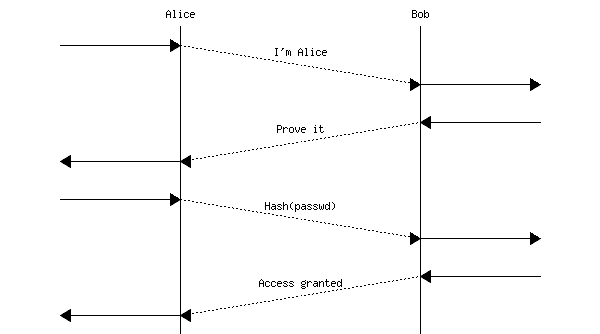Since the hash function cannot be inverted, an eavesdropper cannot extract Alice’s password by simply observing the data exchanged. However, Alice’s real password is not the objective of an attacker. The main objective for Mallory is to be authenticated as Alice. If Mallory can capture Hash(passwd), he can simply replay this data, without being able to invert the hash function. This is called a replay attack.

To counter this replay attack, we need to ensure that Alice never sends the same information twice to Bob. A possible mode of operation is shown below.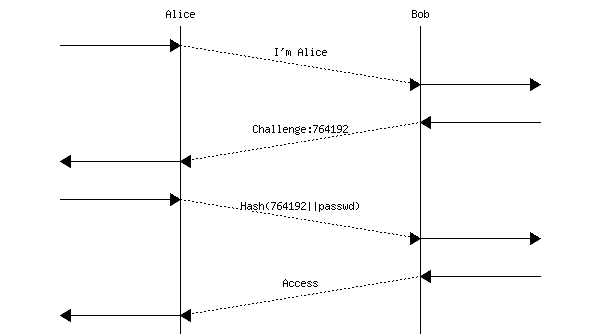To authenticate herself, Alice sends her user identifier to Bob. Bob replies with a random number as a challenge to verify that Alice knows the shared secret (i.e. her password). Alice replies with the result of the computation of a hash function (e.g. SHA-1) over a string that is the concatenation between the random number chosen by Bob and Alice’s password. The random number chosen by Bob is often called a nonce since this is a number that should only be used once. Bob performs the same computation locally and can check the message returned by Alice. This type of authentication scheme has been used in various protocols. It prevents replay attacks. If Eve captures the messages exchanged by Alice and Bob, she cannot recover Alice’s password from the messages exchanged since hash functions are non-invertible. Furthermore, she cannot replay the hashed value since Bob will always send a different nonce.

Unfortunately, this solution forces Bob to store Alice’s password in clear. Any breach in the security of Bob’s computer would reveal Alice’s password. Such breaches unfortunately occur and some of them have led to the dissemination of millions of passwords.

A better approach would be to authenticate Alice without storing her password in clear on Bob’s computer. For this, Alice computes a hash chain as proposed by Lamport in [Lamport1981]. A hash chain is a sequence of applications of a hash function (H) on an input string. If Alice’s password is P, then her 10 steps hash chain is : $$H(H(H(H(H(H(H(H(H(H(P))))))))))$$. The result of this hash chain will be stored on Bob’s computer together with the value 10. This number is the maximum number of remaining authentications for Alice on Bob’s computer. To authenticate Alice, Bob sends the remaining number of authentications, i.e. 10 in this example. Since Alice knows her password, P, she can compute $$H^9(P)=H(H(H(H(H(H(H(H(H(P)))))))))$$ and send this information to Bob. Bob computes the hash of the value received from Alice ($$H(H^9(P))$$) and verifies that this value is equal to the value stored in his database. It then decrements the number of authorized authentications and stores $$H^9(P)$$ in his database. Bob is now ready for the next authentication of Alice. When the number of authorized authentications reaches zero, the hash chain needs to be reinitialized. If Eve captures $$(H^n(P))$$, she cannot use it to authenticate herself as Alice on Bob’s computer because Bob will have decremented its number of authorized authentications. Furthermore, given that hash functions are not invertible, Eve cannot compute $$H^{n-1}(P)$$ from $$H^{n}(P)$$.

The two protocols above prevent eavesdropping attacks, but not man-in-the-middle attacks. If Mallory can intercept the messages sent by Alice, he could force her to reveal $$H^n(P)$$ and then use this information to authenticate as Alice on Bob’s computer. In practice, hash chains should only be used when the communicating users know that there cannot be any man-in-the-middle on their communication.

Public key cryptography provides another possibility to allow Alice to authenticate herself on Bob’s computer. Assume again that Alice and Bob know each other from previous encounters. Alice knows Bob’s public key ($$Bob_{pub}$$) and Bob also knows Alice’s key ($$Alice_{pub}$$). To authenticate herself, Alice could send her user identifier. Bob would reply with a random number encrypted with Alice’s public key : $$E_p(Alice_{pub},R)$$. Alice can decrypt this message to recover R and sends $$E_p(Bob_{pub},R)$$. Bob decrypts the nonce and confirms that Alice knows $$Alice_{priv}$$. If an eavesdropper captures the messages exchanged, he cannot recover the value R which could be used as a key to encrypt the information with a secret key algorithm. This is illustrated in the time sequence diagram below.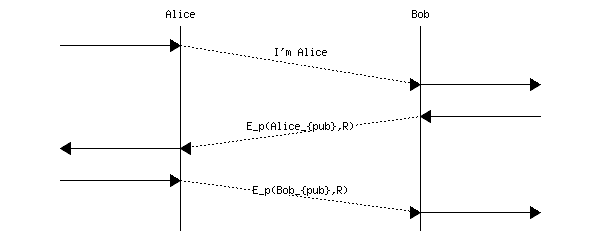A drawback of this approach is that Bob is forced to perform two public key computations : one encryption to send the random nonce to Alice and one decryption to recover the nonce encrypted by Alice. If these computations are costly from a CPU viewpoint, this creates a risk of Denial of Service Attacks were attackers could try to access Bob’s computer and force it to perform such costly computations. Bob is more at risk than Alice in this situation and he should not perform complex operations before being sure that he is talking with Alice. An alternative is shown in the time sequence diagram below.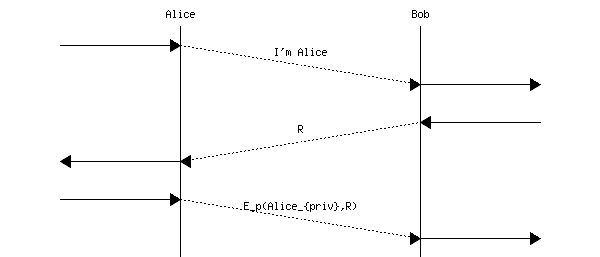Here, Bob simply sends a random nonce to Alice and verifies her signature. Since the random nonce and the signature could be captured by an eavesdropper, they cannot be used as a secret key to encrypt further data. However Bob could propose a secret key and send it encrypted with Alice’s public key in response to the signed nonce that he received.

The solution described above works provided that Bob and Alice know their respective public keys before communicating. Otherwise, the protocol is not secure against man-in-the-middle attackers. Consider Mallory sitting in the middle between Alice and Bob and assume that neither Alice nor Bob knows the other’s public key.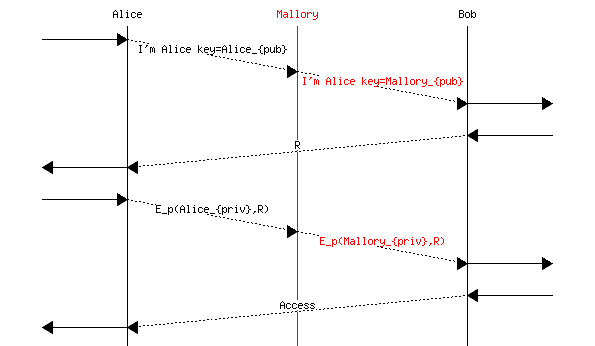In the above example, Alice sends her public key, ($$Alice_{pub}$$), in her first message together with her identity. Mallory intercepts the message and replaces Alice’s key with his own key, ($$Mallory_{pub}$$). Bob replies with a nonce, R. Alice then signs the random nonce to prove that she knows $$Alice_{priv}$$. Mallory discards the information and instead computes $$E_p(Mallory_{priv},R)$$. Bob now thinks that he is discussing with Alice while Mallory sits in the middle.

There are situations where symmetric authentication is required. In this case, each user must perform some computation with his/her private key. A possible exchange is the following. Alice sends her certificate to Bob. Bob replies with a nonce, $$R1$$, and provides his certificate. Alice encrypts $$R1$$ with her private key and generates a nonce, $$R2$$. Bob verifies Alice’s computation and encrypts $$R2$$ with his private key. Alice verifies the computation and both have been authenticated.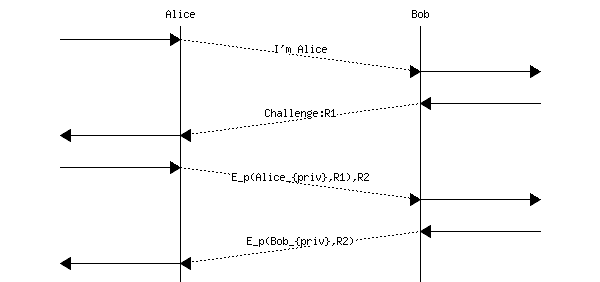The protocol described above works, but it takes a long time for Bob to authenticate Alice and for Alice to authenticate Bob. A faster authentication could be the following.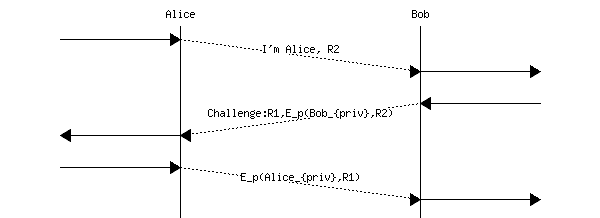Alice sends her random nonce, $$R2$$. Bob signs $$R2$$ and sends his nonce : $$R1$$. Alice signs $$R1$$ and both are authenticated.

Now consider that Mallory wants to be authenticated as Alice. The above protocol has a subtle flaw that could be exploited by Mallory. This flaw can be exploited if Alice and Bob can act as both client and server. Knowing this, Mallory could operate as follows. Mallory starts an authentication with Bob faking himself as Alice. He sends a first message to Bob including Alice’s identity.In this exchange, Bob authenticates himself by signing the $$RA$$ nonce that was sent by Mallory. Now, to authenticate as Alice, Mallory needs to compute the signature of nonce $$RB$$ with Alice’s private key. Mallory does not know Alice’s key, but he could exploit the protocol to force Alice to perform the required computation. For this, Mallory can start an authentication to Alice as shown below.In this example, Mallory has forced Alice to compute $$E_p(Alice_{priv},RB)$$ which is the information required to finalize the first exchange and be authenticated as Alice. This illustrates a common problem with authentication schemes when the same information can be used for different purposes. The problem comes from the fact that Alice agrees to compute her signature on a nonce chosen by Bob (and relayed by Mallory). This problem occurs if the nonce is a simple integer without any structure. If the nonce includes some structure such as some information about Alice and Bob’s identities or even a single bit indicating whether the nonce was chosen by a user acting as a client (i.e. starting the authentication) or as a server, then the protocol is not vulnerable anymore.

To cope with some of the above mentioned problems, public-key cryptography is usually combined with certificates. A certificate is a data structure that includes a signature from a trusted third party. A simple explanation of the utilization of certificates is to consider that Alice and Bob both know Ted. Ted is trusted by these two users and both have stored Ted’s public key : $$Ted_{pub}$$. Since they both know Ted’s key, he can issue certificates. A certificate is mainly a cryptographic link between the identity of a user and his/her public key. Such a certificate can be computed in different ways. A simple solution is for Ted to generate a file that contains the following information for each certified user :

• his/her identity

• his/her public key

• a hash of the entire file signed with Ted’s private key

Then, knowing Ted’s public key, anyone can verify the validity of a certificate. When a user sends his/her public key, he/she must also attach the certificate to prove the link between his/her identity and the public key. In practice, certificates are more complex than this. Certificates will often be used to authenticate the server and sometimes to authenticate the client.

A possible protocol could then be the following. Alice sends $$Cert(Alice_{pub},Ted)$$. Bob replies with a random nonce.Until now, we have only discussed the authentication problem. This is an important but not sufficient step to have a secure communication between two users through an insecure network. To securely exchange information, Alice and Bob need to both :

• mutually authenticate each other

• agree on a way to encrypt the messages that they will exchange

Let us first explore how this could be realized by using public-key cryptography. We assume that Alice and Bob have both a public-private key pair and the corresponding certificates signed by a trusted third party : Ted.

A possible protocol would be the following. Alice sends $$Cert(Alice_{pub},Ted)$$. This certificate provides Alice’s identity and her public key. Bob replies with the certificate containing his own public key : $$Cert(Bob_{pub},Ted)$$. At this point, they both know the other public key and could use it to send encrypted messages. Alice would send $$E_p(Bob_{pub},M1)$$ and Bob would send $$E_p(Alice_{pub},M2)$$. In practice, using public key encryption techniques to encrypt a large number of messages is inefficient because these cryptosystems require a large number of computations. It is more efficient to use secret key cryptosystems for most of the data and only use a public key cryptosystem to encrypt the random secret keys that will be used by the secret key encryption scheme.

## Key exchange¶

When users want to communicate securely through a network, they need to exchange information such as the keys that will be used by an encryption algorithm even in the presence of an eavesdropper. The most widely used algorithm that allows two users to safely exchange an integer in the presence of an eavesdropper is the one proposed by Diffie and Hellman [DH1976]. It operates with (large) integers. Two of them are public, the modulus, p, which is prime and the base, g, which must be a primitive root of p. The communicating users select a random integer, $$a$$ for Alice and $$b$$ for Bob. The exchange starts as :

• Alice selects a random integer, $$a$$ and sends $$A=g^{a} \mod p$$ to Bob

• Bob selects a random integer, $$b$$ and sends $$B=g^{b} \mod p$$ to Alice

• From her knowledge of $$a$$ and $$B$$, Alice can compute $$Secret=B^{a} \mod p= (g^{b} \mod p) ^{a} \mod p=g^{a \times b} \mod p$$

• From is knowledge of $$b$$ and $$A$$, Bob can compute $$Secret=A^{b} \mod p=(g^{a} \mod p) ^{b} \mod p=g^{a \times b} \mod p$$

The security of this protocol relies on the difficulty of computing discrete logarithms, i.e. from the knowledge of $$A$$ (resp. $$B$$), it is very difficult to extract $$\log(A)=\log(g^{a} \mod p)=a$$ (resp. $$\log(B)=\log(g^{b} \mod p)=b$$).

An example of the utilization of the Diffie-Hellman key exchange is shown below. Before starting the exchange, Alice and Bob agree on a modulus ($$p=23$$) and a base ($$g=5$$). These two numbers are public. They are typically part of the standard that defines the protocol that uses the key exchange.

• Alice chooses a secret integer : $$a=8$$ and sends $$A= g^{a} \mod p= 5^{8} \mod 23=16$$ to Bob

• Bob chooses a secret integer : $$b=13$$ and sends $$B= g^{b} \mod p=5^{13} \mod 23=21$$ to Alice

• Alice computes $$S_{A}=B^{a} \mod p= 21^{8} \mod 23=3$$

• Bob computes $$S_{B}=A^{b} \mod p= 16^{13} \mod 23=3$$

Alice and Bob have agreed on the secret information $$3$$ without having sent it explicitly through the network. If the integers used are large enough and have good properties, then even Eve who can capture all the messages sent by Alice and Bob cannot recover the secret key that they have exchanged. There is no formal proof of the security of the algorithm, but mathematicians have tried to solve similar problems with integers during centuries without finding an efficient algorithm. As long as the integers that are used are random and large enough, the only possible attack for Eve is to test all possible integers that could have been chosen by Alice and Bob. This is computationally very expensive. This algorithm is widely used in security protocols to agree on a secret key.

Unfortunately, the Diffie-Hellman key exchange alone cannot cope with man-in-the middle attacks. Consider Mallory who sits in the middle between Alice and Bob and can easily capture and modify their messages. The modulus and the base are public. They are thus known by Mallory as well. He could then operate as follows :

• Alice chooses a secret integer and sends $$A= g^{a} \mod p$$ to Mallory

• Mallory generates a secret integer, $$m$$ and sends $$M=g^{m} \mod p$$ to Bob

• Bob chooses a secret integer and sends $$B=g^{b} \mod p$$ to Mallory

• Mallory computes $$S_{A}=A^{m} \mod p$$ and $$S_{B}=B^{m} \mod p$$

• Alice computes $$S_{A}=M^{a} \mod p$$ and uses this key to communicate with Mallory (acting as Bob)

• Bob computes $$S_{B}=M^{b} \mod p$$ and uses this key to communicate with Mallory (acting as Alice)

When Alice sends a message, she encrypts it with $$S_{A}$$. Mallory decrypts it with $$S_{A}$$ and encrypts the plaintext with $$S_{B}$$. When Bob receives the message, he can decrypt it by using $$S_{B}$$.

To safely use the Diffie-Hellman key exchange, Alice and Bob must use an authenticated exchange. Some of the information sent by Alice or Bob must be signed with a public key known by the other user. In practice, it is often important for Alice to authenticate Bob. If Bob has a certificate signed by Ted, the authenticated key exchange could be organized as follows.

• Alice chooses a secret integer : $$a$$ and sends $$A= g^{a} \mod p$$ to Bob

• Bob chooses a secret integer : $$b$$, computes $$B= g^{b} \mod p$$ and sends $$Cert(Bob,Bob_{pub},Ted), E_p(Bob_{priv},B)$$ to Alice

• Alice checks the signature (with $$Bob_{pub}$$) and the certificate and computes $$S_{A}=B^{a} \mod p$$

• Bob computes $$S_{B}=A^{b} \mod p$$

This prevents the attack mentioned above since Mallory cannot create a fake certificate and cannot sign a value by using Bob’s private key. Given the risk of man-in-the-middle attacks, the Diffie-Hellman key exchange mechanism should never be used without authentication.

Footnotes

1